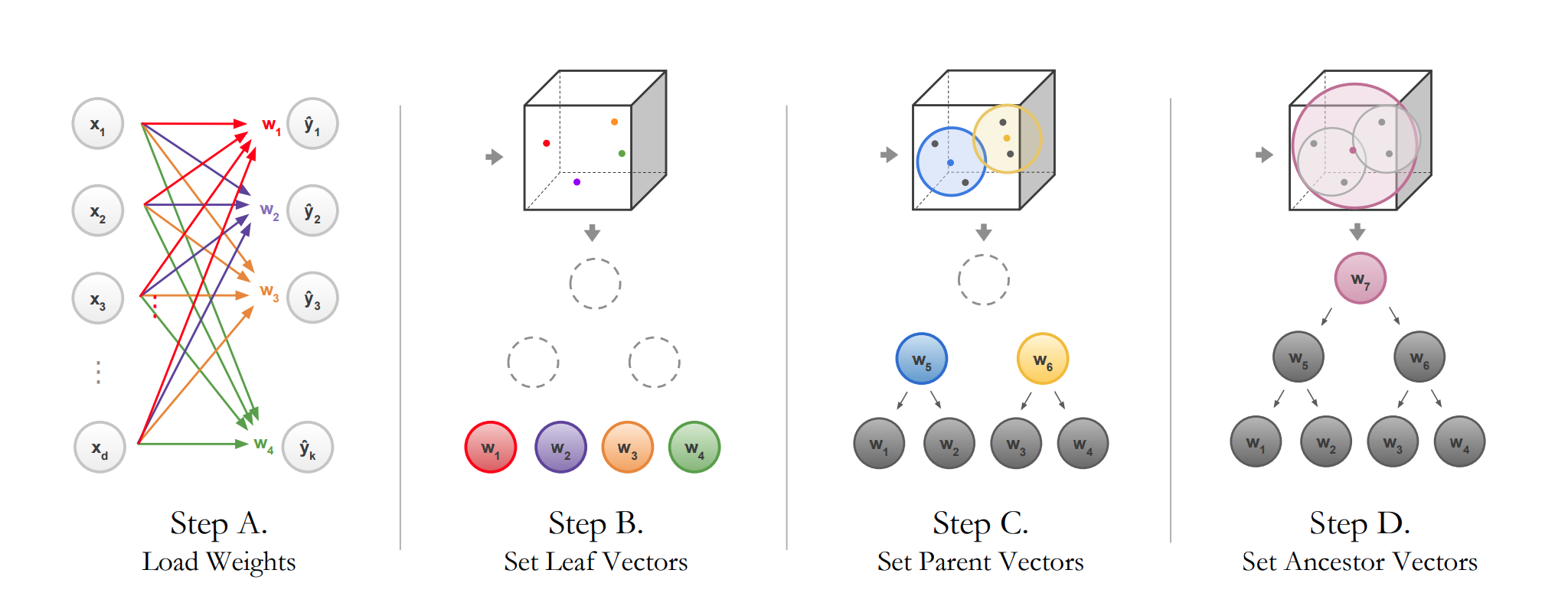# NBDT: Neural-Backed Decision Trees¶

linkStep A: Load the weights of the last fully-connected layer.
Step B: Load the wights as different nodes.
Step C, D: The wight of parent is the average weight of its children.

The nodes in same layer form a classifier. Parents' labels come from WordNet, for example, if the leaf node is dog, its parent node can be animal.

After step D, they fine-tuned their models, with a loss $\mathcal{L}$,

$$\mathcal{L}=\beta_{t} \underbrace{\text { CROSSENTROPY }\left(\mathcal{D}_{\text {pred }}, \mathcal{D}_{\text {label }}\right)}_{\mathcal{L}_{\text {original }}}+\omega_{t} \underbrace{\text { CROSSENTROPY }\left(\mathcal{D}_{\text {nbdt }}, \mathcal{D}_{\text {label }}\right)}_{\mathcal{L}_{\text {soft }}}$$

$D_{nbdt}$ is the defined in section 3.1 of this paper.In :
%%sh
NBDT https://static.toiimg.com/thumb/msid-67586673,width-800,height-600,resizemode-75,imgsize-3918697,pt-32,y_pad-40/67586673.jpg
NBDT https://cdn.jpegmini.com/user/images/slider_puffin_jpegmini.jpg

not enough values to unpack (expected 2, got 0)
Prediction: cat // Decisions: animal (99.27%), chordate (99.38%), carnivore (99.36%), cat (99.81%)
not enough values to unpack (expected 2, got 0)
Prediction: bird // Decisions: animal (97.51%), chordate (98.96%), vertebrate (99.05%), bird (99.76%)

stty: stdin isn't a terminal
stty: stdin isn't a terminal